Custom Search
If you want to discuss more on this or other issues related to Physics, feel free to leave a message on my Facebook page.

## Tuesday, November 24, 2009

### What is a vector and how to add vector?

A vector is an object that has a magnitude, size or length and direction. It is generally represented by an arrow that starts from a initial point (tail) to an end at a terminal point (tip).

Here the initial point is A and the terminal point is B. The magnitude is the length of the arrow. The longer the arrow the greater the magnitude of the vector. And the direction of the arrow is the direction of the vector.However in physics the direction of the vector is often represented by an angle θ1 with respect to the horizontal or an angle θ2 with respect to the vertical.

A vector is named by using the initial and terminal point as shown in the diagram below. Hence the vector shown is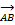.The vector can also be name by using a single letter that may be bold or using the arrow on the letter as shown in the diagram below. Hence the vector can be named either using B or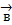.We are now going to see how vectors are subtracted, added and multiplied.

In order to show you how to add vectors we are going to use vectors that horizontal.

Let us have a look at two vectors A and B. Vector A has magnitude 4 and vector B has magnitude 2.Here it is important to note that as vector A has a magnitude twice that of vector B then the length of vector A must be twice that of B.

A + BTo add vector A and B you place the tail of vector B on the tip of vector A. The result is as shown in the diagram. The two vectors drawn as such is A + B.

As you can see the length of the two vectors together is 6.

Hence the A + B = 6

What would happen if the two vectors are as shown below.Then A + B is obtained by placing the tail of vector B on the tip of vector A as shown below.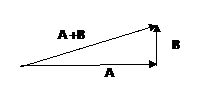The vector that starts from the tail of A to the tip of B is the vector (A + B). But as you can see here the magnitude of the vector A + B must be calculated using Pythagoras theorem.

If the two vectors A and B are not at right angle to each other, then the angles a and b  that the two vectors make to the vertical or the horizontal must be known as shown in the diagram below.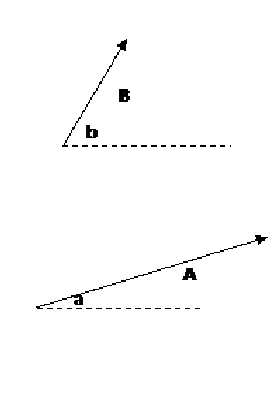Hence to add these two vectors we just place the tail of vector B on the tip of vector A as shown below. Then the vector that starts from the tail of vector A to the tip of vector B is the vector (A + B)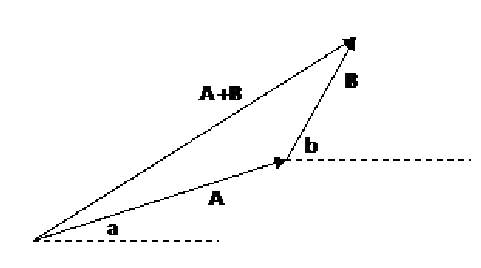In this example the two vectors A and B have angle a and b relative to the horizontal respectively. Hence using the two angles a and b, the angle between the two vectors can be found and as a result the vector A + B can be found using the cosine rule.

#### 1 comment:

1.This is really good you should go further like teach how to use measuring instruments like micrometer screw gauges and vernier calipers,etc
good jb thoe.:-)